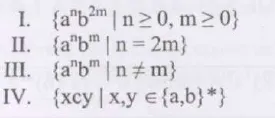# Theory of Computation MCQs

## MCQs on Theory of Computation

Solve Theory of Computation Multiple-Choice Questions to prepare better for GATE. Learn more about Theory of Computation and Theory of Computation MCQs by checking notes, mock tests, and previous years’ question papers. Gauge the pattern of MCQs on Theory of Computation by solving the ones that we have compiled below for your practice:

## Theory of Computation Multiple-Choice Questions

1. In the finite automaton with minimum state deterministic that accepts a given language L={w | w ε {0,1} *, the total number of 0s as well as 1s in w that would be divisible by 3 & 5, respectively} would have:

a. 9 states

b. 10 states

c. 11 states

d. 15 states

2. If we consider an arbitrary NFA (non-deterministic finite automaton) with N states in total, the maximum number of states that are there in an equivalent DFA (minimised) is at least:

a. N!

b. 2N

c. 2^N

d. N^2

3. Which one of these given regular expressions isn’t equivalent to this regular expression:

(m + n + o) *

a. (m*n* + o*)*

b. ((mn)* + o*)*

c. (m*n*o*)*

d. (m* + n* + o*)*

4. Consider that we have a G ambiguous grammar along with its D disambiguated version. If the language that is recognized by these two grammars is denoted by L(G) and L(D), then which one of these would be true?

a. L (D) = L (G)

b. L (D) ⊂ L (G)

c. L (D) is empty

d. L (D) ⊃ L (G)

Answer: (a) L (D) = L (G)

5. If you consider a regular expression r, in which r = (11 + 111)* over Ʃ = {0, 1}, then the number of states in minimal DFA and NFA respectively are:

a. DFA – 4, NFA – 3

b. DFA – 3, NFA – 3

c. DFA – 3, NFA – 4

d. DFA – 4, NFA – 4

Answer: (a) DFA – 4, NFA – 3

6. The language that a Pushdown Automation accepts in which the stack stays limited to about 10 items is described best as:

a. Recursive

b. Deterministic Context Free

c. Regular

d. Context Free

7. The C language is a:

a. Regular language

b. Context free language

c. Language parsable fully by a Turing machine only

d. Context sensitive language

8. Consider the language given below:

{a^m b^n C^(m+n) | m, n ≥ 1}

It is a ___________ language.

a. regular

b. not context free but context sensitive

c. not context sensitive but type-0

d. not regular but context-free

Answer: (d) not regular but context-free

9. Which of these is a regular set?a. I

b. IV

c. I and III

d. I and IV

10. Consider the following languages:

L1 = {0^i1^j | i != 2j}

L2 = {0^i1^j | i = 2j+1}

L3 = {0^i1^j | i = j}

L4 = {0^i1^j | i != j}

Which of these is/are context free:

a. Only L3

b. Only L3 & L2

c. Only L4 & L3

d. All LA, L2, L3, and L4

Answer: (d) All LA, L2, L3, and L4

11. The L= {0^i21^i | i≥0 } language over the {0,1, 2} alphabet is:

a. a CFL but not a deterministic CFL

b. a regular language

c. is recursive as well as a deterministic CFL

d. not recursive

Answer: (c) is recursive as well as a deterministic CFL

12. If G is the CFG, r is the total number of rightmost derivations, l is the total number of leftmost derivations, as well as P refers to the total number of parse trees, then assume that r, l, and P are computed for some given particular string. Here, for a given ‘G’ CFG and given ‘w’ string, what is the relation between all three of these?

a. r ≤ P ≥ l

b. r = P = l

c. r ≥ P ≤ l

d. None

Answer: (b) r = P = l

13. When L and L’ happen to be recursively enumerable, here L is:

a. context-free

b. regular

c. recursive

d. context-sensitive

14. Which of these problems given below is undecidable?

a. The Ambiguity problem for the CFGs

b. The Membership problem for the CFGs

c. The Equivalence problem for the FSAs

d. The Finiteness problem for the FSAs

Answer: (a) The Ambiguity problem for the CFGs

15. If L refers to the language that is generated by S – OSO/00, then which of these is true?

a. L is not O but regular

b. L is regular but not context-free

c. L is not context-free

d. L = O

Answer: (b) L is regular but not context-free

Keep learning and stay tuned to get the latest updates on the GATE Exam along with GATE MCQs, GATE Eligibility Criteria, GATE Syllabus for CSE (Computer Science Engineering), GATE Notes for CSE, GATE CSE Question Paper, and more.